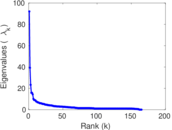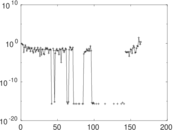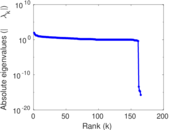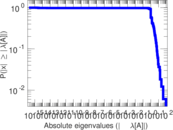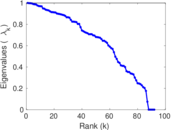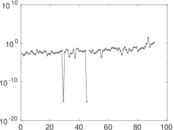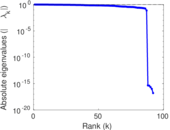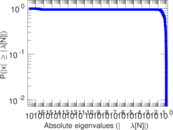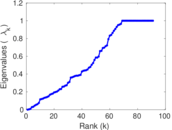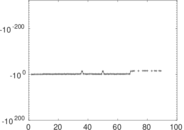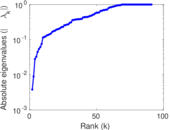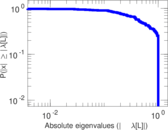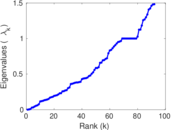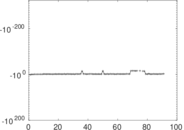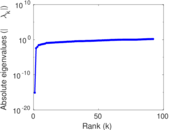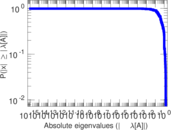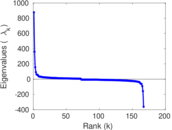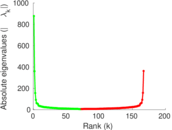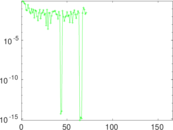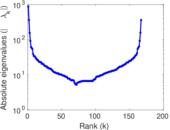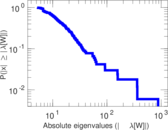# Wiktionary edits (ks)

This is the bipartite edit network of the Kashmiri Wiktionary. It contains users and pages from the Kashmiri Wiktionary, connected by edit events. Each edge represents an edit. The dataset includes the timestamp of each edit.

 Code `mks` Internal name `edit-kswiktionary` Name Wiktionary edits (ks) Data source http://dumps.wikimedia.org/ AvailabilityDataset is available for download Consistency checkDataset passed all tests Category Authorship network Dataset timestamp 2017-10-20 Node meaning User, article Edge meaning Edit Network formatBipartite, undirected Edge typeUnweighted, multiple edges Temporal dataEdges are annotated with timestamps

## Statistics

 Size n = 888 Left size n1 = 167 Right size n2 = 721 Volume m = 1,884 Unique edge count m̿ = 1,156 Wedge count s = 40,762 Claw count z = 1,835,512 Cross count x = 72,026,329 Square count q = 7,842 4-Tour count T4 = 228,408 Maximum degree dmax = 508 Maximum left degree d1max = 508 Maximum right degree d2max = 48 Average degree d = 4.243 24 Average left degree d1 = 11.281 4 Average right degree d2 = 2.613 04 Fill p = 0.009 600 77 Average edge multiplicity m̃ = 1.629 76 Size of LCC N = 694 Diameter δ = 15 50-Percentile effective diameter δ0.5 = 5.146 82 90-Percentile effective diameter δ0.9 = 9.213 90 Median distance δM = 6 Mean distance δm = 5.698 80 Gini coefficient G = 0.688 041 Balanced inequality ratio P = 0.226 380 Left balanced inequality ratio P1 = 0.173 567 Right balanced inequality ratio P2 = 0.295 117 Relative edge distribution entropy Her = 0.844 568 Power law exponent γ = 3.462 78 Tail power law exponent γt = 2.191 00 Tail power law exponent with p γ3 = 2.191 00 p-value p = 0.985 000 Left tail power law exponent with p γ3,1 = 1.751 00 Left p-value p1 = 0.186 000 Right tail power law exponent with p γ3,2 = 3.091 00 Right p-value p2 = 0.060 000 0 Degree assortativity ρ = −0.209 913 Degree assortativity p-value pρ = 5.603 74 × 10−13 Spectral norm α = 92.063 1 Algebraic connectivity a = 0.003 808 25 Spectral separation |λ1[A] / λ2[A]| = 2.345 22 Controllability C = 561 Relative controllability Cr = 0.635 334

## Plots

### Fruchterman–Reingold graph drawing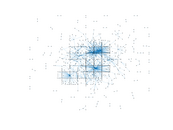### Degree distribution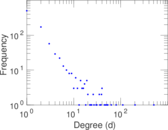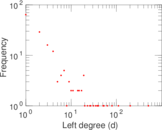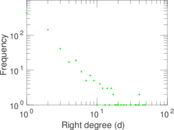### Cumulative degree distribution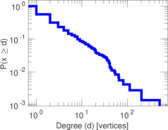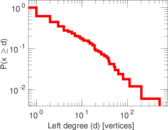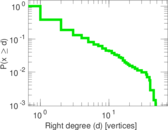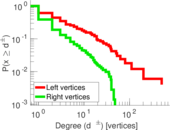### Lorenz curve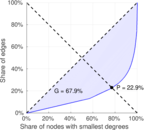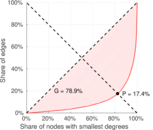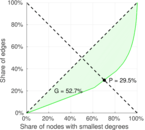### Spectral distribution of the adjacency matrix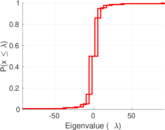### Spectral distribution of the normalized adjacency matrix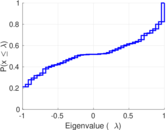### Spectral distribution of the Laplacian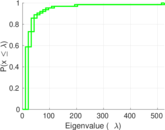### Spectral graph drawing based on the adjacency matrix### Spectral graph drawing based on the Laplacian### Spectral graph drawing based on the normalized adjacency matrix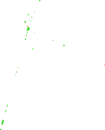### Degree assortativity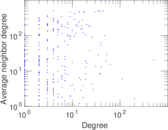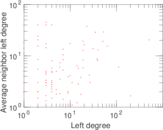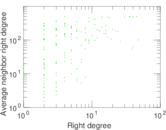### Zipf plot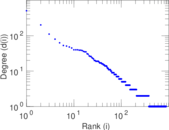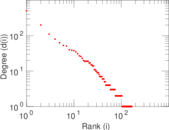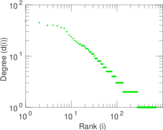### Hop distribution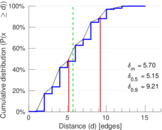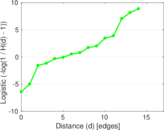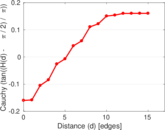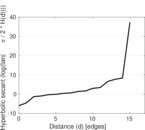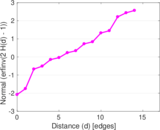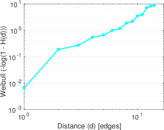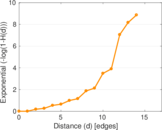### Double Laplacian graph drawing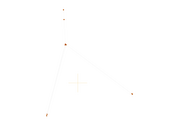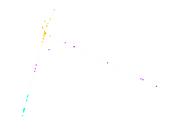### Delaunay graph drawing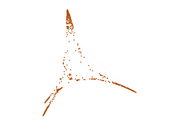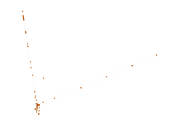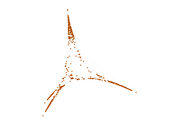### Edge weight/multiplicity distribution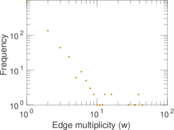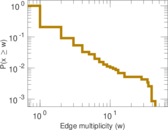### Temporal distribution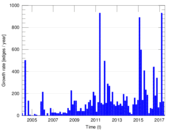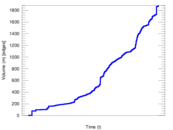### Temporal hop distribution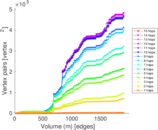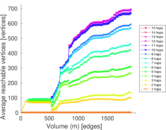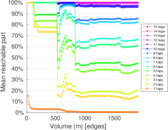### Diameter/density evolution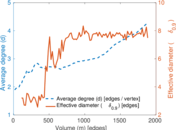### Matrix decompositions plots